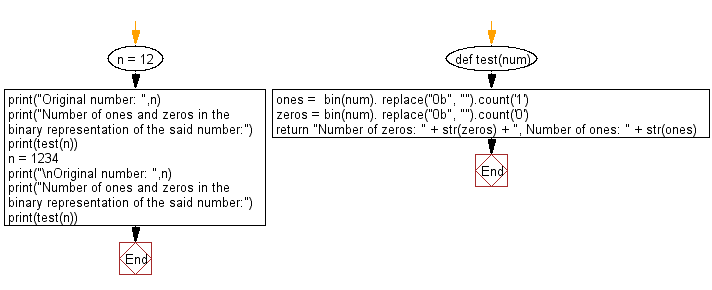﻿ Python: Count number of zeros and ones in the binary representation of a given integer - w3resource

# Python: Count number of zeros and ones in the binary representation of a given integer

## Python Basic - 1: Exercise-131 with Solution

Write a Python program to count the number of zeros and ones in the binary representation of a given integer.

Sample Solution

Python Code:

``````def test(num):
ones =  bin(num). replace("0b", "").count('1')
zeros = bin(num). replace("0b", "").count('0')
return "Number of zeros: " + str(zeros) + ", Number of ones: " + str(ones);

n = 12;
print("Original number: ",n);
print("Number of ones and zeros in the binary representation of the said number:");
print(test(n));
n = 1234;
print("\nOriginal number: ",n);
print("Number of ones and zeros in the binary representation of the said number:");
print(test(n));
``````

Sample Output:

```Original number:  12
Number of ones and zeros in the binary representation of the said number:
Number of zeros: 2, Number of ones: 2

Original number:  1234
Number of ones and zeros in the binary representation of the said number:
Number of zeros: 6, Number of ones: 5
```

Flowchart:Python Code Editor:

Have another way to solve this solution? Contribute your code (and comments) through Disqus.

What is the difficulty level of this exercise?

Test your Programming skills with w3resource's quiz.

﻿